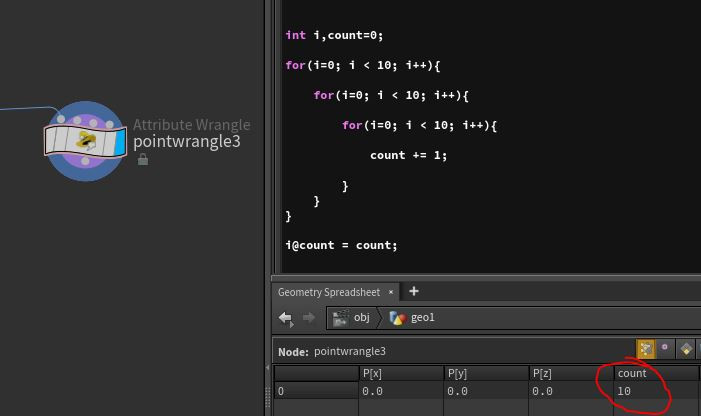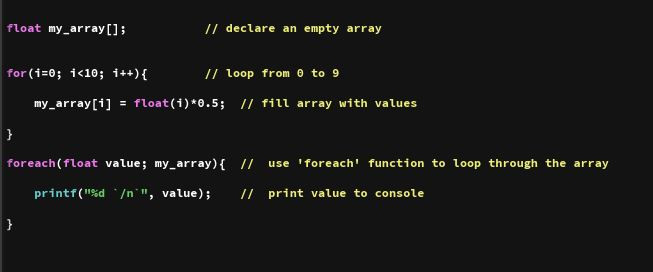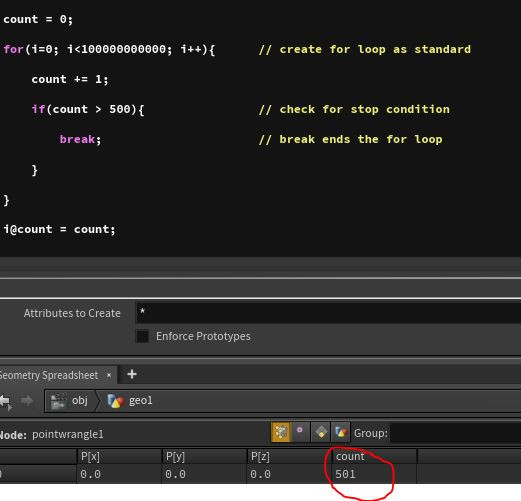Search
•Matt Norris

# Introduction to VEX part 3 (Syntax / Structure)

Updated: Feb 25, 2020That's right people, it's time don your favourite hacker shades and declare some flipping variables!!!

As I said earlier I'm not going to be going through all of the basics of programming but I am going to quickly blitz through the basic language structure of VEX. I'll add another caveat in here, I learned coding with Python and have a distinctly pythonic formatting style, VEX is actually a very forgiving language and I've seen people format their wrangles in all sorts of ways so don't take it for granted that my formatting style is anything other than my own personal preference. Also I find the vast majority of VEX work I do is in wrangles, working at the geometry level, so unless specifically stated otherwise I'll be working in a wrangle style way.

I'll apologise in advance for this post being quite dry, it's definitely meant more as a reference than a tutorial, something to dip in and out of if you can't quite remember how to format a while loop or cast a component of a matrix, if you get bored reading this by all means skip ahead to something more interesting!

The absolute very best place to find details on VEX syntax and structure is, surprisingly, the Houdini VEX docs

# Declaring variablesHere we go, straight into it. Unfortunately the formatting options for the blog site I use a bit limited so what I'll do is upload a screen grab of my code in Houdini, then follow that with a horrifically formatted wall of text you can copy and paste if needed.Horrifically formatted wall of text:

1. int my_integer = 0; // declaring an integer variable with an explicit value

2. int my_other_integer; // declaring an integer variable without a value

3. int not_another_integer = my_integer * 10; // declaring an integer with an implicit value

4. float my_float = float(my_integer); // declaring a float by re-casting an integer variable

5. float second_float = 0.11111; //declaring a float variable with an explicit value

6. float something_clever = v@P.x; // declaring a float by reading a point attribute

7. vector my_vector = {1.0,2.0,3.0}; // declare a vector variable using an explicit value

8. vector new_vector = set(my_float, second_float, something_clever); //using the set() function to declare a vector variable with implicit values.

9. vector position = v@P; // declaring a vector variable by reading a point attribute

10. matrix3 my_matrix = {1,0,0,0,1,0,0,0,1}; // declaring a 3x3 matrix variable using explicit variables

11. matrix3 ident_mat = ident(); // declaring an identity matrix using the ident() function

12. matrix3 rot_mat = set(my_vector, new_vector, position); // using the set() function to declare a matrix variable from implicit vector variables.

13. int my_int_array[] = {0, 1, 2, 3, 4, 5, 6}; // declare an integer array from explicit values

14. float my_float_array[] = {0.0, 0.1, 0.2, 0.3, 0.4}; // declare a float array from explicit values

15. vector my_vector_array[]; // declare an uncast, empty vector array

16. my_vector_array = my_vector; // set vector array value using vector variable

So, there we go, everything you need to declare variables, well mostly, as I said this is not meant to be a 100% complete reference to VEX, for that check out this link. Instead it's just a quick guide to demonstrate formatting and syntax structure.

# Loopingscreengrab:As you can see VEX includes support for both for and while loops, one potential gotcha for people used to working with more sophisticated programming languages is that VEX doesn't seem to handle local variable declaration very nicely. Here's an example:As you can see in this example 'i' only gets declared once, each loop is using the same variable to run it's iteration so when 'i' reaches 10 in the 3rd loop the 2nd and 1st loop break too. So only 10 iterations are run.In this example we are declaring new versions of 'i' in each for loop, this works, the value for count is correct however VEX throws a warning and to my mind this is a bit untidy. Which is why in my first example I use distinct iterators for each of my nested loops i, j ,k or i1 ,i2 ,i3. Again this is just a preference but it feels to me like a more precise way of organising my code.

Again here's some code for you!

1. int i, j, k, count=0; // declare uncast integer variables 'i', 'j' and 'k', declare second integer variable 'count' with explicit value

2. for(i=0; i<10; i+=1){ // declare first loop, (x; y; z) where x = initial iterator value, y = iterator stop condition, z = iterator increment

3. for(j=10; j>0; j-=1){ // declare second nested for loop, a new iterator must be used as VEX doesn't differentiate between local variables

4. k = 0; // initialise value for 'k'

5. while(k < 10){ // create while loop with stop condition

6. k+=1; // increment while loop iterator

7. count +=1; // increment previously initialised 'count' variable

8. }

9. }

10. }

11. i@count = count; // bind export 'count' as an integer attribute to the points

VEX has some other iteration methods that are more situational such as 'pciterate' and 'forpoints' but they are dedicated iteration methods that only work with certain types of objects/data so I'll avoid mentioning them for now, however I will mention 'foreach' which is a looping method designed to work with arrays and as such can be quite useful.As you can see 'foreach' doesn't need an iterator or a stop condition it will just loop over every item in the array, returning the value of the item to the variable you declare as the first argument in the 'foreach' function.

code:

1. float my_array[]; // declare an empty array

2. for(i=0; i<10; i++){ // loop from 0 to 9

3. my_array[i] = float(i)*0.5; // fill array with values

4. }

5. foreach(float value; my_array){ // use 'foreach' function to loop through the array

6. printf("%d `/n`", value); // print value to console

7. }

# If Else StatementsPretty basic stuff, no messing about:if statement
1. int x = 0, y = 1, z; // declare some variables

2. if(x < y){ // all comparisons need to be done in parenthesis ()

3. z = 4; // the procedure to be run if comparison is true

4. }if else statement

1. if(y < z){

2. z = 3;

3. }else{ // else statements directly after if statement works as expected

4. z = 2;

5. }

A full list of the comparison operators for VEX can be found in the reference docs here.

# Flow ControlBy combining loops and if statements VEX allows you to setup some fairly complex flow control operations, these can be useful for adding a bit of optimisation when looping over very large data sets:The break function can be used to exit a loop early, when combined with an if statement you can create faster loops!

code:

1. count = 0;

2. for(i=0; i<100000000000; i++){ // create for loop as standard

3. count += 1;

4. if(count > 500){ // check for stop condition

5. break; // break ends the for loop

6. }

7. }Continue is a bit more complex, when continue is invoked it tells the loop to immediately stop processing the current iteration and skip to the next one. In this example we can see that the 2 lines directly after the continue statement only get executed once, when 'count' hits 500.

code:

1. count = 0;

2. int check = 0;

3. for(i=0; i<100000000000; i++){ // create for loop as standard

4. count += 1;

5. if(count<500)continue; // the if statement checks for a stop condition, if the stop condition isn't met the for loop will 'continue'

6. check += 12345;

7. break; // break ends the for loop

8. }

9. i@count = count;

10. i@check = check;

# Accessing Geometry Object AttributesI'll be honest, I struggled to find an appropriate gif for this topic.

This is the bread and butter of what we do, manipulating geometry level data, whether it's animating point positions or procedurally building geometry or setting per-point sim attribute. What makes Houdini stand apart from any other software I have used is the access it gives an artist to the fundamental building blocks of your geometry.Again I'm not going to list every single type of attribute VEX can handle (because there's a shed load of them!) the docs have a complete list here however they all follow this same basic structure.

code:

1. i@my_int = 2; // explicit cast of integer attribute

2. f@my_float = 3.147; // explicit cast of float attribute

3. v@my_vector = {1.1, 2.2, 3.3}; // explicit cast of vector attribute using {}

4. p@my_quaternion = {1,0,0,0}; // explicit cast of quaternion attribute using {}

5. 3@my_rotation_matrix = set(v@my_vector, v@my_vector * 2, v@my_vector * 0.5); // implicit cast of 3x3 matrix attribute using set() function

6. 4@my_transform_matrix = ident(); // creation of 4x4 identity matrix using ident() function

7. i[]@my_int_array = array(i@my_int, 3, 45, 87); // implicit cast of integer array using the array() function

8. f[]@my_float_array = array(f@my_float, @Frame, @Time); // implicit cast of float array using the array() function

9. int my_int = i@my_int; // casting an integer variable from an integer attribute

10. float my_float = f@my_float; // casting a float variable from a float attribute

11. float my_vector_X = v@my_vector.x; // casting a float variable from the 'x' component of a vector attribute

12. float my_rotation_matrix_XY = 3@my_rotation_matrix.xy; // casting a float variable from the 'xy' component of a 3x3 matrix attribute

Almost there, just one last topic on this reference section and then I promise I'm going to actually write some proper code!

# Channel Referencing

Just to reiterate an important point, when you're writing wrangles and working on your geometry with VEX you cannot directly access data outside of your current point in the script, outside of the node or wrangle you are working on. Think of each node as a specific function that gets run in a specific order, the variables you create inside the wrangle only exist in that node, and likewise the only data that wrangle has access to is the data that is being piped into one of it's inputs.

So this begs the question, what if I need to access a global variable? What if I need to access a parameter from a different node?

Well, in a wrangle, VEX can read parameters on the wrangle you are writing, this allows you to pipe into the VEX code you are writing pretty much any type of data which you can't directly access inside the wrangle.code:

1. int my_integer = chi("my_integer"); // cast integer variable from integer parameter

2. float my_float = chf("my_float"); // cast float variable from float parameter

3. vector my_vector = chv("my_vector"); // cast vector variable from vector parameter

4. string my_string = chs("my_string"); // cast string variable from string parameter

5. matrix3 my_rot_mat = ch3("my_rot_mat"); // cast matrix3 variable from matrix3 parameter

6. i@frame = my_integer; // bind integer variable to integer attribute

7. f@pi = my_float; // bind float variable to float attribute

8. v@centroid = my_vector; // bind vector variable to vector attribute

9. s@hipfile = my_string; // bind string variable to string attributeAlright, nice job people. That covers the basics and should be a handy reference for how to do the simple stuff.

In my next post I promise I'll actually write some proper flipping code!